## Example Questions

### Example Question #35 : Trigonometry

Find the range of: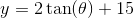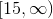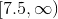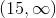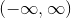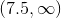Explanation:

The functionis related to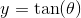.  The range of the tangent function is.

The range ofis unaffected by the amplitude and the y-intercept.  Therefore, the answer is.

### Example Question #36 : Trigonometry

What is the range of the trigonometric fuction defined by: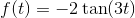?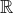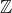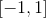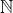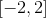Explanation:

For tangent and cotangent functions, the range is always all real numbers.

### Example Question #37 : Trigonometry

What is the range of the given trigonometric function: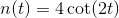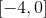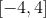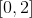The range of a function is every value that the funciton's results take. For tangent and cotangent, the function spans fromand so the range is: